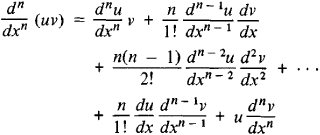# Leibniz Formula

The following article is from The Great Soviet Encyclopedia (1979). It might be outdated or ideologically biased.

## Leibniz Formula

a formula for the n th derivative of the product of two functions in terms of the derivatives of the factors:The formula was reported by G. von Leibniz in a letter to Johann Bernoulli in 1695. The Leibniz formula facilitates the finding of derivatives of higher orders.